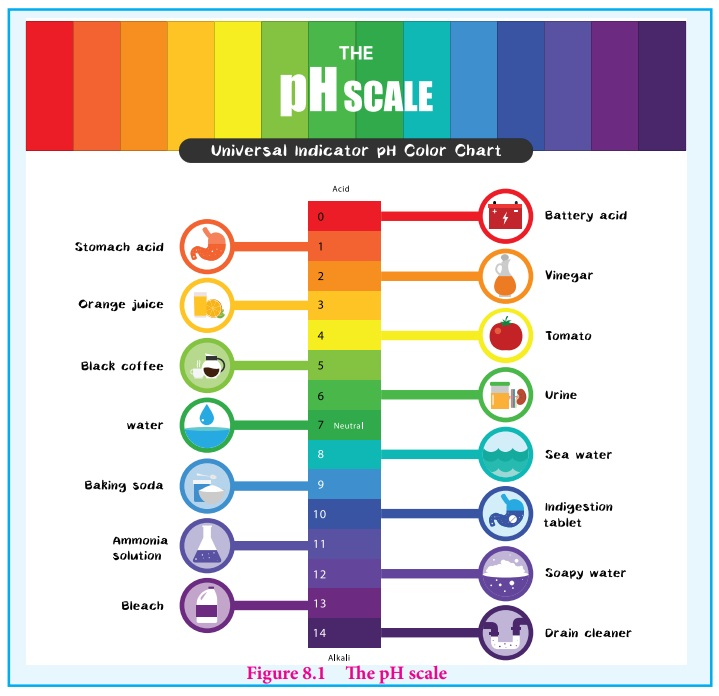Home | | Chemistry 12th Std | The pH scale

# The pH scale

pH of a solution is defined as the negative logarithm of base 10 of the molar concentration of the hydronium ions present in the solution.

The pH scale

We usually deal with acid / base solution in the concentration range 10-1 to 10-7M . To express the strength of such low concentrations, Sorensen introduced a logarithmic scale known as the pH scale. The term pH is derived from the French word ŌĆśPurissance de hydrogeneŌĆÖ meaning, the power of hydrogen. pH of a solution is defined as the negative logarithm of base 10 of the molar concentration of the hydronium ions present in the solution.

pH = - log 10[H3O+ ]      .....(8.5)

The concentration of H3O+ in a solution of known pH can be calculated using the following expression.

[H3O+] = 10-pH (or) [H3O+] = antilog of (-pH)   .....(8.6)

Similarly, pOH can also be defined as follows

pOH = - log 10[OH-]     .....(8.7)

As discussed earlier, in neutral solutions, the concentration of [H3O+ ] as well as [OH+] is equal to 1 ├Ś 10-7 M at 25┬║C . The pH of a neutral solution can be calculated by substituting this H3O+ concentration in the expression (8.5)

pH = - log10 [H3O+]

= - log 10 10-7

= (-7)(-1) log1010= +7 (1)= 7    { [ log1010 =1] }

Similary, we can calculate the pOH of a neutral solution using the expression (8.7), it is also equal to 7.

The negative sign in the expression (8.5) indicates that when the concentration of [H3O+ ] increases the pH value decreases. For example, if the [H3O+ ] increases from to 10-7 to10-5 M , the pH value of the solution decreases from 7 to 5. We know that in acidic solution, [H3O+ ] > [OH- ] , i.e., [H3O+ ] > 10-7 . Similarly in basic solution [H3O+ ] < 10-7. So, we can conclude that acidic solution should have pH value less than 7 and basic solution should have pH value greater than 7.

## Relation between pH and pOH

A relation between pH and pOH can be established using their following definitions

pH = -log10[H3O+]    .....(8.5)

pOH = - log10[OH-]    .....(8.7)pH + pOH = - log10[H3O+] - log10[OH-]

= - ( log10[H3O+] + log10[OH-])

pH+pOH = -log10[H3O+][OH-]       [log a+logb = logab]

We know that [H3O+][OH-]=Kw

ŌćÆ pH + pOH = -log10 Kw

ŌćÆ pH+pOH = pKw        [pKw = -log10 Kw]

at 25┬║ C, the ionic product of water, Kw =1 ├Ś 10-14

pKw = - log1010-14 = 14 log1010

= 14

Ōł┤ (8.7) ŌćÆ Ōł┤ At 25┬║ C, pH + pOH= 14

### Example 8.2

Calculate the pH of 0.001M HCl solutionH3O+ from the auto ionisation of H2O (10-7M) is negligible when compared to the H3O+ from 10-3M HCl.

Hence [H3O+ ]= 0.001 mol dmŌĆō3

pH = -log10 [H3O+]

= -log10 (0.001)

= -log10 (10-3 ) = 3

Note: If the concentration of the acid or base is less than 10ŌĆō6 M, the concentration of H3O+ produced due to the auto ionisation of water cannot be negleted and in such cases

[H3O+] = 10-7(from water) + [H3O+] (from the acid)

similarly, [OH-] = 10-7 M (from water) + [OH-] (from the base)

### Example 8.3

Calculate pH of 10-7 M HCl

If we do not consider [H3O+ ] from the ionisation of H2O,

then [H3O+ ] = [HCl] = 10-7M

i.e., pH = 7, which is a pH of a neutral solution. We know that HCl solution is acidic whatever may be the concentration of HCl i.e, the pH value should be less than 7. In this case the concentration of the acid is very low (10-7M) Hence, the H3O+ (10-7M) formed due to the auto ionisation of water cannot be neglected.

so, in this case we should consider [H3O+ ] from ionisation of H 2O

[H3O+ ] = 10-7 (from HCl) + 10-7 (from water)

= 10-7 (1+1)

= 2 ├Ś10-7

pH = -log10[H3O+ ]

= - log 2 - (-7).log10 10

= 7-log 2

= 7-0.3010 = 6.6990

= 6.70

### Evaluate yourself ŌĆō 6

a. Calculate pH of 10-8M H2 SO4

b. Calculate the concentration of hydrogen ion in moles per litre of a solution whose pH is 5.4

c. Calculate the pH of an aqueous solution obtained by mixing 50ml of 0.2 M HCl with 50ml 0.1 M NaOHIn this case the concentration of H2SO4 is very low and hence [H3O+ ] from water cannot be neglected

Ōł┤[H3O+] = 2 ├Ś10-8 (from H2SO4 ) + 10-7

(from water)

= 10-8 (2+10)

= 12├Ś10-8 = 1.2 ├Ś10-7

pH = - log10[H3O+]

= - log10 (1.2 ├Ś 10-7)

= 7 - log101.2

= 7 -0.0791

= 6.9209

pH of the solution = 5.4

[H3O+] = antilog of (-pH)

= anitlog of (-5.4)

= antilog of (-6 + 0.6) = 6.6

= 3.981├Ś10-6

i.e., 3.98 ├Ś 10-6 mol dm-3

No of moles of HCl = 0.2├Ś 50 ├Ś 10-3 = 10 ├Ś 10-3

No of moles of NaOH = 0.1 ├Ś 50 ├Ś 10-3 = 5 ├Ś 10-3

No of moles of HCl after mixing = 10 ├Ś 10-3 - 5 ├Ś 10-3

= 5 ├Ś10-3

after mixing total volume = 100mL

Ōł┤ Concentration of HCl in moles per litre =  5├Ś10-3mole /  100├Ś10-3L

[H3O+] = 5 ├Ś10-2 M

pH = - log (5 ├Ś 10-2 )

= 2 - log 5

= 2 - 0.6990

= 1.30

Tags : Ionic Equilibrium | Chemistry , 12th Chemistry : UNIT 8 : Ionic Equilibrium
Study Material, Lecturing Notes, Assignment, Reference, Wiki description explanation, brief detail
12th Chemistry : UNIT 8 : Ionic Equilibrium : The pH scale | Ionic Equilibrium | Chemistry